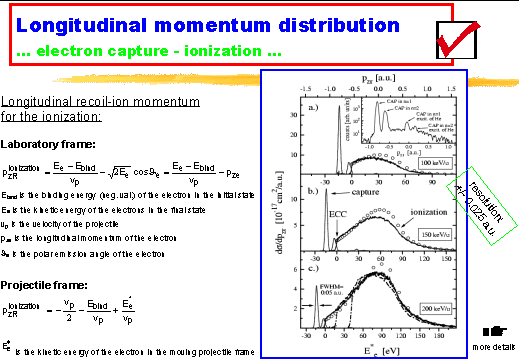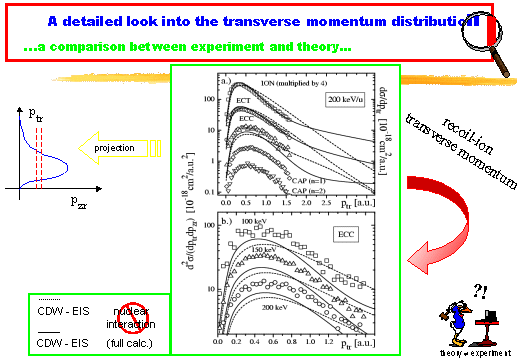Abrupt rise of the longitudinal recoil ion momentum distribution for ionizing collisions

 ABSTRACT We report on the experimental observation of an abrupt rise in the longitudinal momentum distribution of recoil-ions created in proton helium collision. The details of this structure can be related to electrons travelling with the velocity of the projectile (electron capture to the continuum ECC). The longitudinal as well as the transverse distribution of the recoil-ions can be explained as a continuation of the momentum distribution from ions resulting from electron capture illustrating the smooth transition from the capture to bound states of the projectile to the ECC. INTRODUCTION The momentum distribution of the fragments in the final state of an atomic collision is the main source of information on the dynamics of such ionization processes. Of particular interest are discrete structures in these momentum distributions, which are directly linked to a particular mechanism or to a special kinematical scenario of how the ionization proceeds. In the momentum distribution of ionized continuum electrons three of such distinct structures are known: 1. A spherical ridge in the velocity space, centered on the projectile velocity, resulting from the Binary Encounter (BE) between the projectile and a quasi-free target electron. And 2. + 3. two peaks at the target- and at the projectile velocity, resulting from very slow electrons ("target-cusp" or Electron Capture to the Target ECT, see , ) and electrons travelling with the projectile without being bound (Electron Capture to the Continuum ECC; see , ,  and references therein and  for a review). In this letter we report on the experimental observation of a new discrete structure in the Helium recoil-ion longitudinal momentum distribution for the reaction: We observe an abrupt rise in the longitudinal recoil-ion momentum distribution that is related to the ECC-processes and which for the first time confirms a theoretical prediction of Rodríguez et al. . EXPERIMENTAL METHOD Details on the experimental technique of Cold Target Recoil Ion Momentum Spectroscopy (COLTRIMS) used for this experiment can be found elsewhere (see  for more detailed information on the experimental setup used for this experiment and  for a review). In brief a precooled supersonic gas jet is crossed with a pulsed ion beam. Up- and downstream of the target gas jet the ion beam is charge state analyzed by electrostatic deflectors. If a projectile is neutralized it is detected by a channel plate detector. An electrostatic field guides the recoil-ions created in the overlap region onto another position-sensitive channel plate detector. From the position of impact on the detector and the time-of-flight the three components of the recoil-ion momentum vector can be determined. With this configuration we were able to measure the recoil-ion momentum distributions for all recoil-ions and simultaneously the ions from single capture reaction only. Since capture leads to clearly separable lines in the recoil-ion momentum distributions, the two measured distributions can be normalized very exactly with respect to each other and the momentum distributions for the pure ionization channel can be obtained just by subtracting these two distributions. RESULTS AND DISCUSSION In Figure 1 the recoil-ion longitudinal momentum distribution for the ionizing (solid line) and the capture-processes (dotted line) for projectile energies of 100, 150 and 200 keV/u are shown. The existence of a minimum value (indicated by ECC in figure 1b) is just a consequence of momentum and energy conservation, i.e. purely a consequence of kinematics. It bears no information on the dynamics or any other detail of the reaction. The physical information lies in the fact in which way the kinematically allowed phase-space is populated. It is remarkable that the cross section has a finite value, i.e. an abrupt rather than a smooth rise. Rodríguez and coworkers have predicted this abrupt rise within a Continuum Distorted Wave Eikonal Initial State (CDW-EIS) calculation (see ). They argued that this is a mirror image of the ECC peak in the electron momentum distribution.Fig. 1: Longitudinal recoil-ion momentum (top-scale) distribution for three different kinetic energies of the projectile (100, 150, 200 keV/u). The solid lines indicate the ionization processes; the dotted curves show the different capture channels and the open circles are results from CDW-EIS theory. A logarithmic plot of the recoil-ion momentum distribution of the capture processes can be seen as an insert in (a). Electron capture in n=1, n=2 of the projectile as well as the same capture channels with simultaneous excitation of the helium target are visible. The scale at the bottom of each spectrum indicates either the kinetic energy of the electrons in the projectile frame , or the bound electron capture energy for . In (c) an overlay of all ionization distributions are shown (dashed-dotted lines) which are normalized to the maximum of the cross section for 200 keV/u. One can also interpret the momentum distribution of figure 1 as a cross section single differential in the electron Energy (Ee) seen from the moving projectile. Ee is indicated by the additional bottom scales in figure 1. In the electron spectra of the laboratory frame the ECC leads to a divergence in the doubly differential cross section. In the projectile frame this divergence is not present, and the distribution shows a smooth transition across the continuum threshold (see figure 1). Thus the capture to highly excited states joins the ionization-continuum continuously across the ECC-edge. The transverse momentum transfer to the recoil-ion as well as the projectile scattering is the result of a subtle interplay in the three-particle interaction. Here theory can help to uncover some of the underlying mechanisms. Therefore, we show in figure 3a+b the experimental transverse momentum distribution for different intervals of the longitudinal momentum in comparison with the result of the full CDW-EIS calculation (solid line) and with the CDW-EIS calculation where the repulsion between the two nuclei has been switched off (dashed line). This figure is just a result of a projection of the doubly differential cross section seen in figure 2. The full calculation in figure 3 shows a narrower ion momentum distribution, which is in much better agreement with all experimental data than the model without the nuclear repulsion. This indicates that the nuclear momentum exchange counteracts the momentum transfer between the electron and the target nucleus, resulting in a smaller mean transverse momentum of the recoil-ion. Large transverse recoil-ion momenta however are still a result of pure nuclear-nuclear scattering. It can be clearly seen that the transverse momentum distribution of the capture and the ECC channel are very similar, again highlighting the smooth transition from capture to ECC. The main contribution to the ionization channel (ION), however peaks at smaller transverse momenta than the ECC and the capture-processes.Fig. 2: The momentum distribution for the recoil-ions of the ionization process for three different kinetic energies of the projectile. The abscissa shows the momentum in the longitudinal direction and the ordinate represents the transverse momentum. The doubly differential cross section d2sigma/(dpzr dptr) is plotted in linear scale. The dash-dot-dot lines (left hand side) indicate the electron capture to the continuum (ECC) while the dashed-dotted lines (right hand side) indicate the target-cusp (ECT). As an example we show the longitudinal momentum distribution of the electrons for the ECT (red box) as a function of the transverse momentum of the electrons.Fig. 3: The transverse recoil-ion momentum distributions for different processes and different intervals of the longitudinal recoil-ion momentum. a.) Squares (ION): Plain ionization (-0.13 < pzr < 0.08 a.u.). Circles (ECT): Target-cusp (0.35 < pzr < 0.45 a.u.). Up triangles (ECC): Cusp (-0.86 < pzr < -0.78 a.u.). Diamonds (CAP): Capture in n=1. Down triangles (CAP): Capture in n=2. The ordinate represents the cross section doubly differential in pzr and ptr for ION and ECT as well as for ECC. The y-axis is single differential in ptr for capture (CAP). The solid lines are results from CDW-EIS theory including the interaction between all three particles; the dashed lines shows CDW-EIS calculation in which the nuclear-nuclear repulsion has been omitted. The kinetic energy of the projectile is 200 KeV/u. b.) ECC processes for three different kinetic energies of the projectile. CONCLUSIONS In conclusion we have found an abrupt rise of the recoil-ion longitudinal momentum distribution linked to the ECC electrons. At very small scattering angles (< 10-4 rad) capture to projectile states of n>1 and ionizing collision leading to the ECC show the same cross section per ion momentum phase space and the same transverse momentum exchange highlighting a smooth transition from capture to ionization in ion atom collisions. REFERENCES  W. Schmitt et al., Phys. Rev. Lett. 81, (1998), p.4337  P.D. Fainstein, Phys. Rev. A 60, (1999), R 741-744  G. B. Crooks et al., Phys. Rev. Lett. 25, (1970), p. 1599  W. Meckbach et al., Phys. Rev. A 24, (1981), p. 1793  J. Macek et al., Phys. Rev. Lett. 46, (1981), p. 1571  N. Stolterfoht et al., Electron Emission in Heavy Ion-Atom Collisions, Springer-Verlag, (1997)  Th. Weber et al., J. Phys. B: At. Mol. Opt. Phys. 33, (2000), p. 3331  V. Rodriguez et al., Phys. Rev. A 52, (1995), R 9  R. Dörner et al., Phys. Rep. 330, Numbers 2-3, (June 2000), pp: 95-192